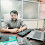# Datatype Concept in C#

3
Datatype Concept in C#:-

It is used to provide a pattern of data and the size of data in memory. The data type will be used into variable, constant, and method declaration.

Data type under variable:-

Datatype variablename=value         int x=10

Data type under constant:-

const Datatype constantname=value    const int y=100

C# has two different types of Datatype

1)  Primitive:-

It Supports all datatype of C and CPP languages.

int     --------->  4byte   (C# has Int16, Int32, Int64)

float  ----------> 4byte, 8 bytes ( float size depends on ox base 32 and 64)

char  -----------> 2byte

string  --------->  Based on no of chars

boolean  ------>  It returns a true or false value using 1 and 0

2)  Derived Datatype:-

This type of Datatype was specially Created by .NET Framework that is common for all .NET Supported programming languages.

object:-   It can contain all types of elements means the object is the common type in the .NET framework. we can assign int, float, char, and String type data under an object.
object o=10;
object o = "hello";

StringBuilder:-  It is used to contain String type data similar to string but StringBuilder is a mutable object means we can dynamically change the value from the actual address.

Class:-   It is used to define the characteristics of an object using data member and member function. it can contain a collection of different types of elements.

Structure:-  It contains a collection of datatype but it is a value type container of c#.

Enumeration:-  It is called Constant Array means we can define a set of values using enum, for example, if we want to define a set of five different colors then we can create an enum set.

Array:-   It can contain elements in Proper Sequence, an array can store more than one element using a single variable.

Collection:-   It Can contain a similar and di-similar type of elements both, it is an enhancement of an array.

DateTime:-  It Can contain Date Time type Values.

Most Important Question of C# for an Interview?

What is the boxing and unboxing concept in C#?
or
What is implicit and explicit conversion in C#?

Boxing means to convert the value of value type elements to reference type, which means if we convert integer type value to object type then it is called boxing.
Boxing is also called implicit because it will convert the data automatically.

Unboxing means converting reference type elements to value type, which means if we convert object type to integer type then it is called the unboxing concept.
Unboxing is also called explicit conversion because it will convert the data manually.

Example of Boxing and Unboxing using the program?

Memory allocation based on data type?

using System;
{
static void Main()
{
object o,a=10;
int b;
o=a;  // int to object
b =(int)o;   // object to int
Console.WriteLine(o + " "+b);

}

}

C# has two different types of memory to store data.

1) Stack Memory:-

It is used to contain the value of int, char, float, double and boolean type elements, it will store data directly hence it is also called direct memory allocation.

2)  Heap Memory:-

It is used to contain the value according to reference String, Object these all are reference type. It is also called Indirect Memory allocation.

ASSIGNMENT?

WAP to calculate simple interest using Object type elements?

WAP to calculate the addition and multiplication of complex numbers?

WAP to calculate Compound Interest?

Tags

1.Solution of Simple Interest Program:-
class SIExample
{
static void Main()
{
object p=25000.23,r=2.2,t=2,si;
/* float p1 = float.Parse(p.ToString());
float r1 = float.Parse(r.ToString());
float t1 = float.Parse(t.ToString());*/
double p1 = Convert.ToDouble(p);
double r1 = Convert.ToDouble(r);
double t1 = Convert.ToDouble(t);
si = (p1*r1*t1)/100;
Console.WriteLine("Result of si is {0}",si);

}

}

2.Solution of Simple Interest Program:-
using System;
using static System.Array;
namespace PracticeS
{
class Program
{
static void Main()
{
int p = 12000, r = 3, t = 12;
int si = (p * r * t)/100;
Console.WriteLine(si);

}
}
}

3.# Program to implement CI

class CI
{
static void Main()
{
double p=50000, r=7, t=2, a;
int n = 12;
r = r / 100;
a = p * Math.Pow(1 + r / n, n * t);

Console.WriteLine("Total amount after int "+a);
Console.WriteLine("Actual IR is " + (a - p));